# Draw the remaining product of the following reaction Cl + 2 HC- CH2- NH2 > H3C-CH2-NH,...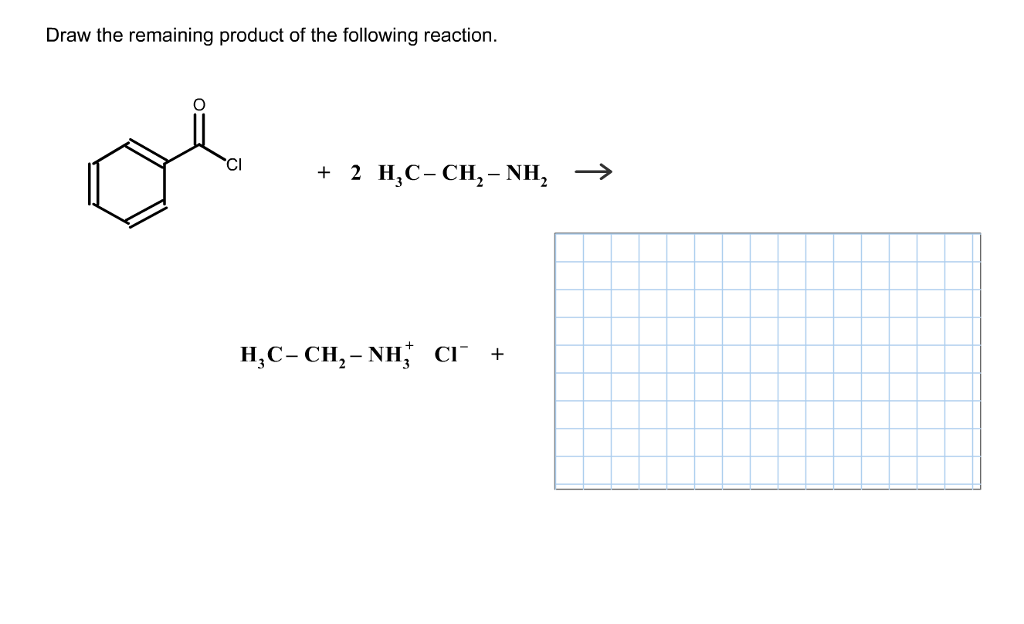Draw the remaining product of the following reaction Cl + 2 HC- CH2- NH2 > H3C-CH2-NH, Cl- +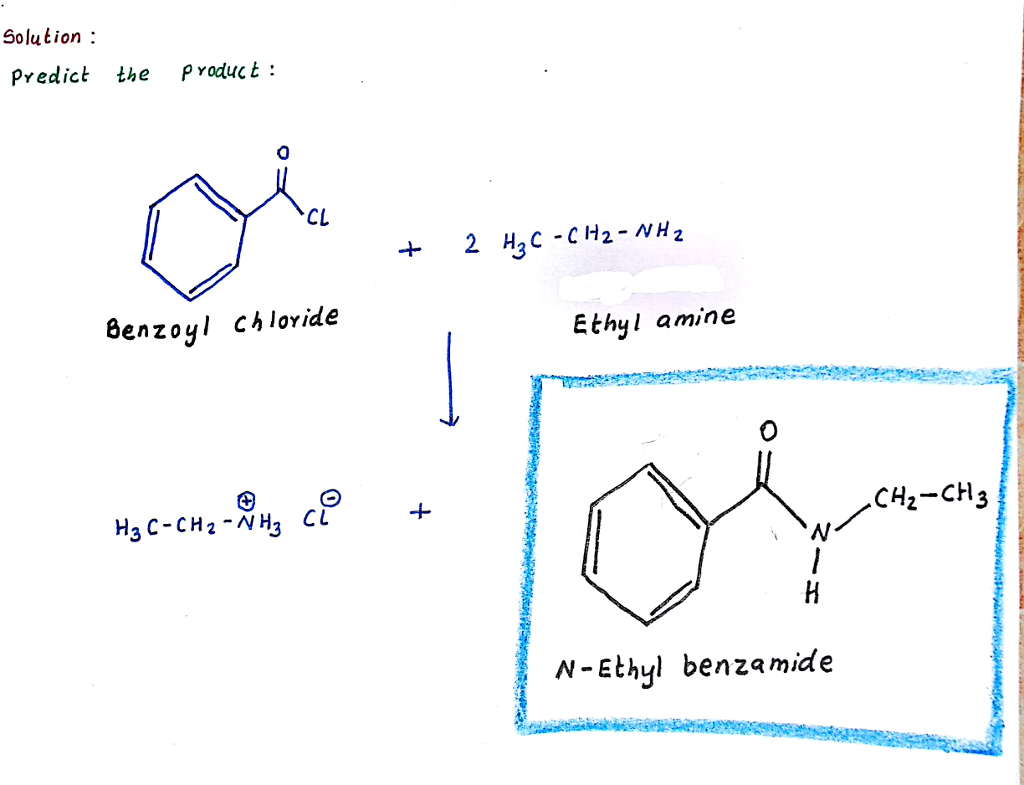#### Earn Coin

Coins can be redeemed for fabulous gifts.

Similar Homework Help Questions
• ### Draw the remaining product of the following reaction CI +2 HC- CH2- NH2 > H,C-CH2-NH CI...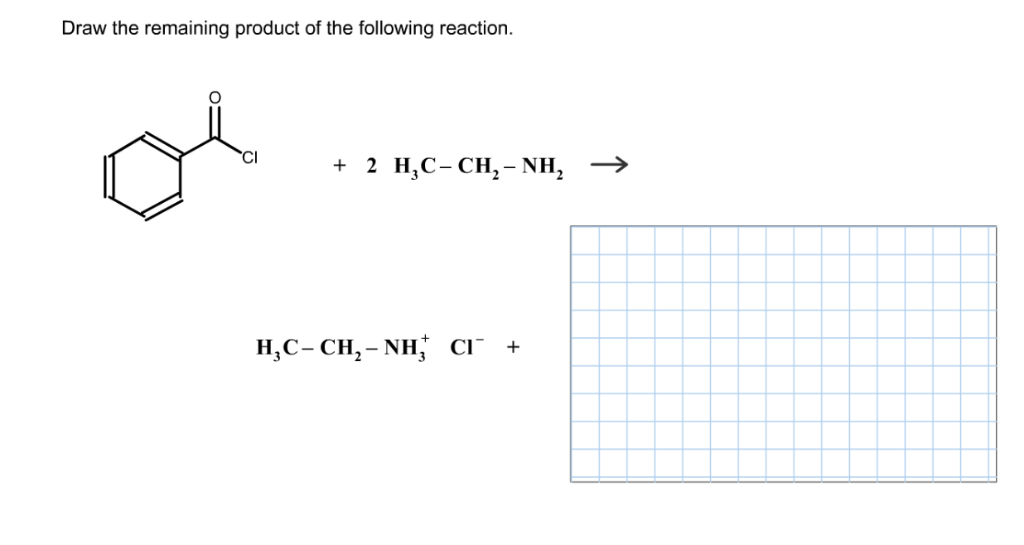Draw the remaining product of the following reaction CI +2 HC- CH2- NH2 > H,C-CH2-NH CI +

• ### Draw the product for the reaction in the space provided: CH3 H3C—=CH2 + HO ">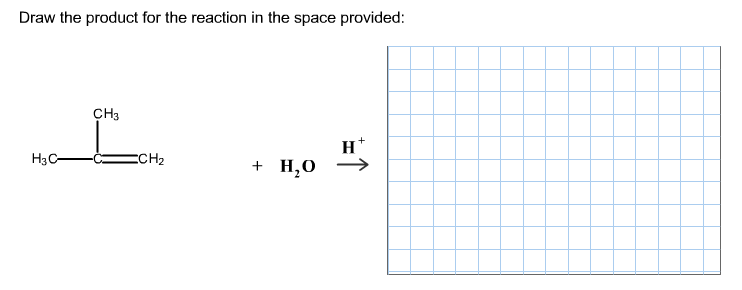Draw the product for the reaction in the space provided: CH3 H3C—=CH2 + HO ">

• ### Draw the product(s) of the following reaction. O=NH ༈.དབས པ ཡང་། + 2 CHỊCH,NH, >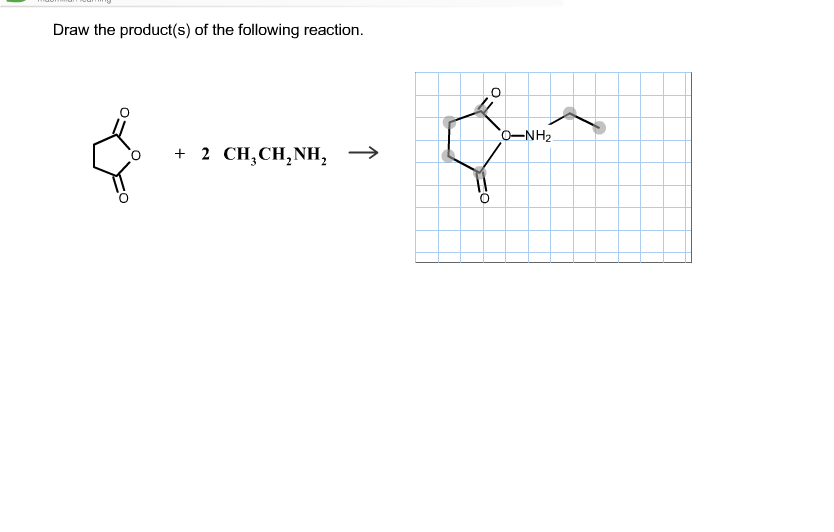Draw the product(s) of the following reaction. O=NH ༈.དབས པ ཡང་། + 2 CHỊCH,NH, >

• ### Resource < Question 12 of 14 > Draw Select H3C NH2 Ho CH-CH2 + HOH- CH-CH2...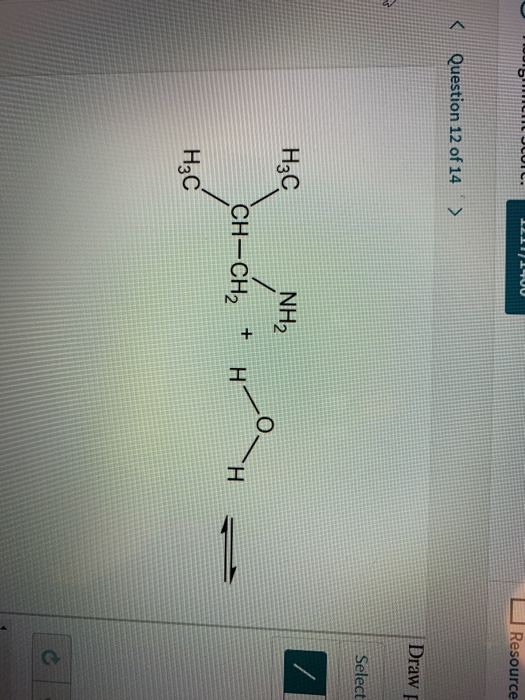Resource < Question 12 of 14 > Draw Select H3C NH2 Ho CH-CH2 + HOH- CH-CH2 H₃C

• ### Draw the final organic product of the reaction. HC OH CH3CH2NH2 > H2O + heat НС....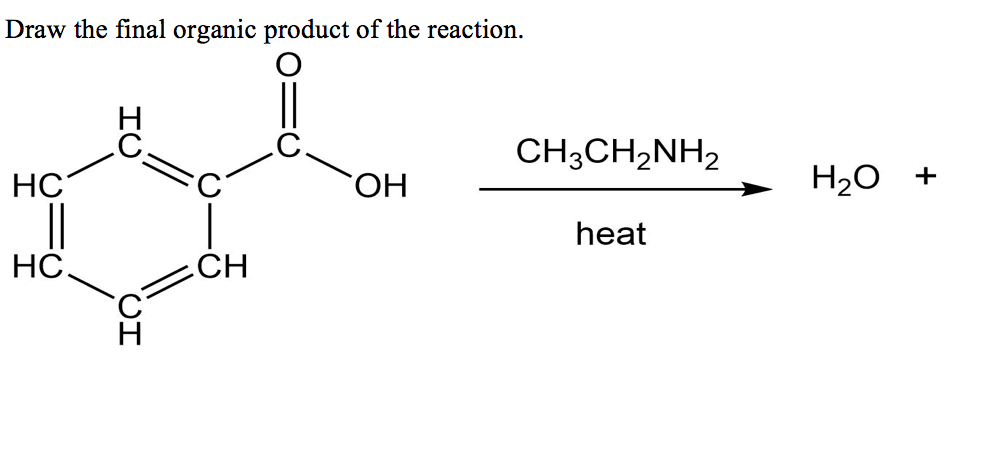Draw the final organic product of the reaction. HC OH CH3CH2NH2 > H2O + heat НС. 2CH IO

• ### KChapter 28 Practice Quiz Chapter 28 Problem 57 17 of 20> Give the product for the...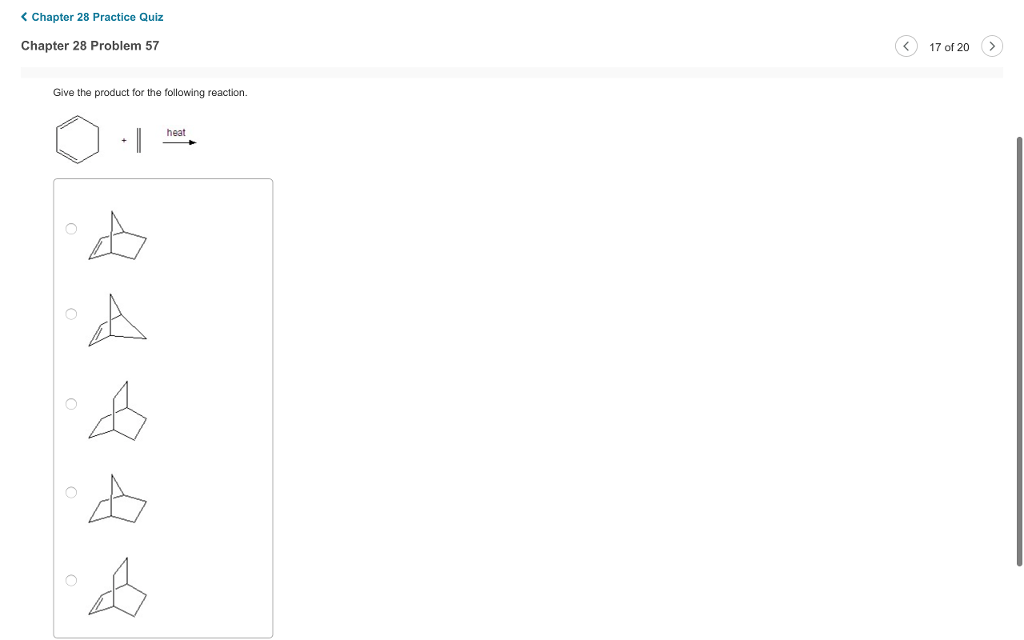KChapter 28 Practice Quiz Chapter 28 Problem 57 17 of 20> Give the product for the following reaction. heat

• ### 6. For the following reaction, + H20* + Cl - > OH + 2H2O Show the...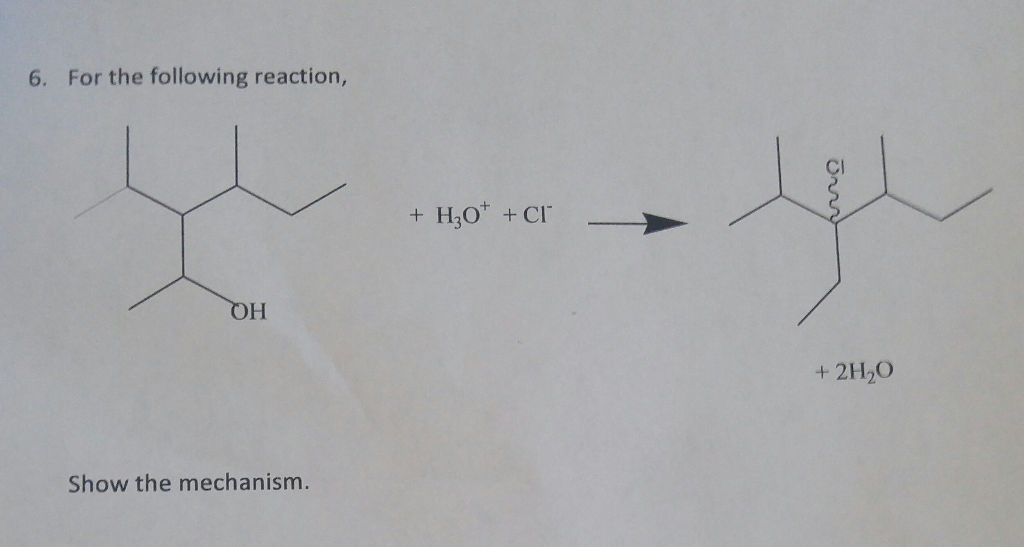6. For the following reaction, + H20* + Cl - > OH + 2H2O Show the mechanism.

• ### Draw the product to the reaction below: -CH3-CH2 CH3 + i H3C—C—HC CH3 + CH3 H3C—CH2-OH...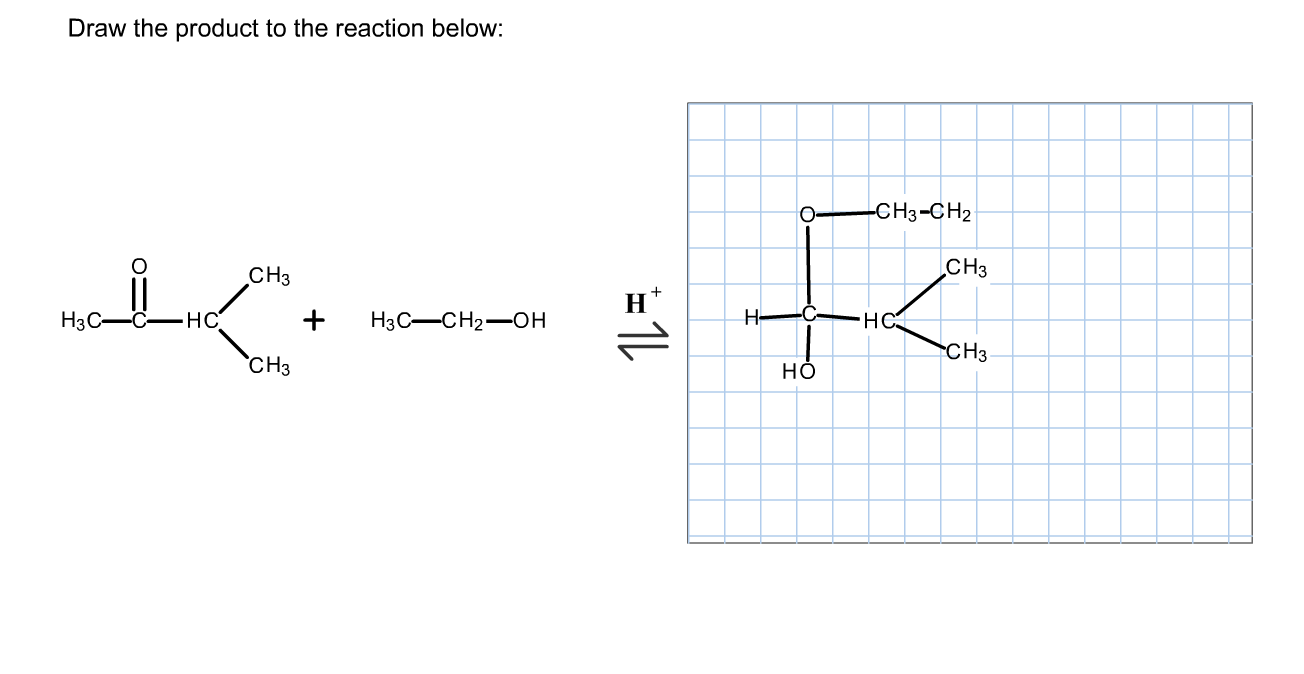Draw the product to the reaction below: -CH3-CH2 CH3 + i H3C—C—HC CH3 + CH3 H3C—CH2-OH CH3

• ### Do the following pairs of structures represent resonance forms that contribute to the same hybrid? :0:...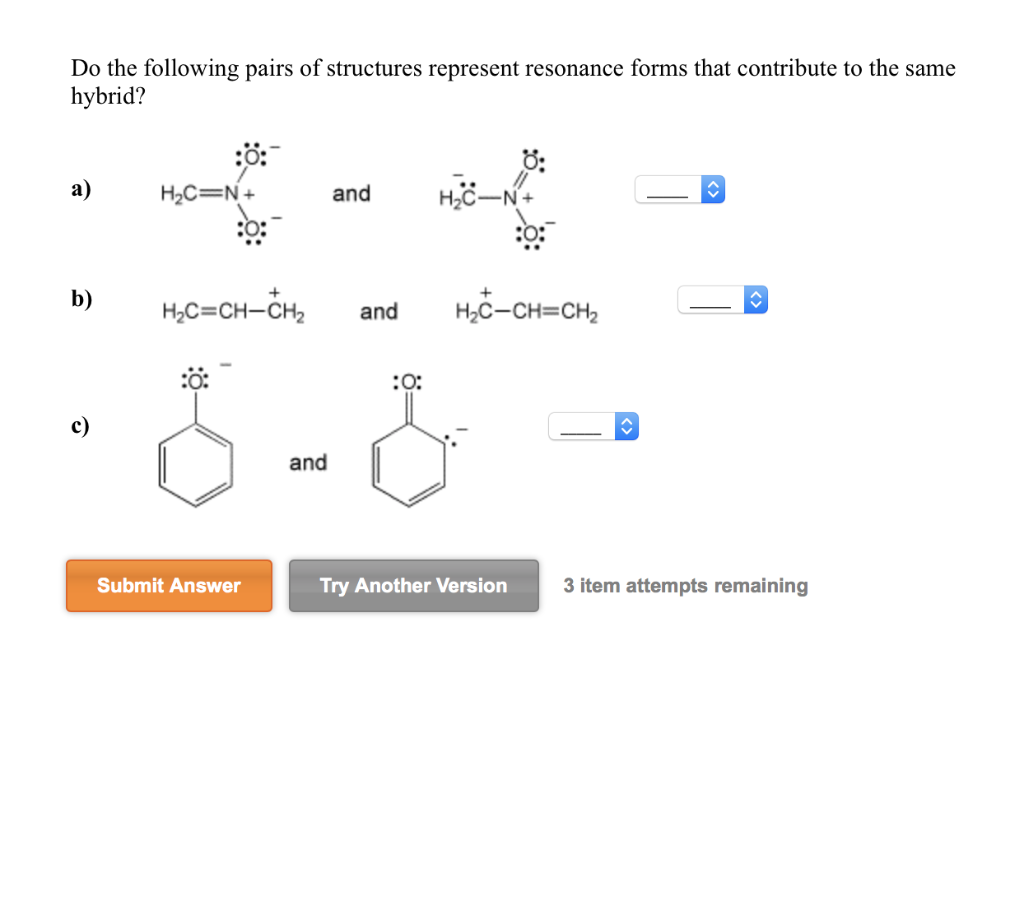Do the following pairs of structures represent resonance forms that contribute to the same hybrid? :0: a) HC=N+ and <> HC-N+ : :o: b) H2C=CH-CH2 and H2C-CH=CH2 .- -- and Submit Answer Submit Answer Try another versions nom atompte romala Try Another Version 3 item attempts remaining

• ### Predict the product(s) of the following reaction 1. KOH 2. CH3CH2CH2Br 3. Но, H2O NH NH2...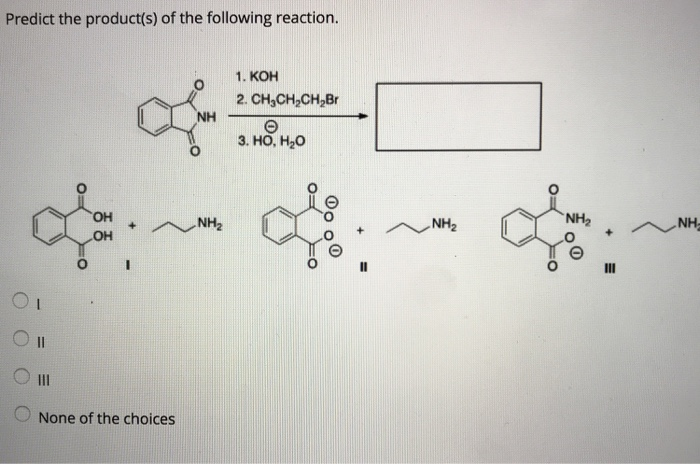Predict the product(s) of the following reaction 1. KOH 2. CH3CH2CH2Br 3. Но, H2O NH NH2 OH O None of the choices What is the correct assignment of the names of the following aromatic amines NH2 NH lpyrr rolidine; Il pyridine; III aniline O 1-pyrrole; Il pyrimidine; III - anisole. I pyrrolidine; Il pyrimidine; III aniline. l pyrrole; Il pyridine; III aniline. Rank the following compounds in order of increasing basicity, putting the least basic compound first NH2 NH2 NH2...

Free Homework App